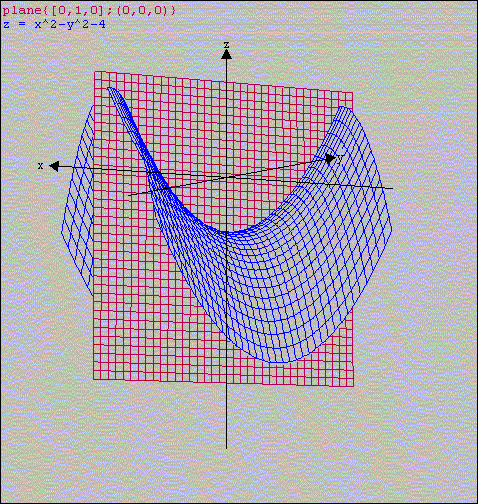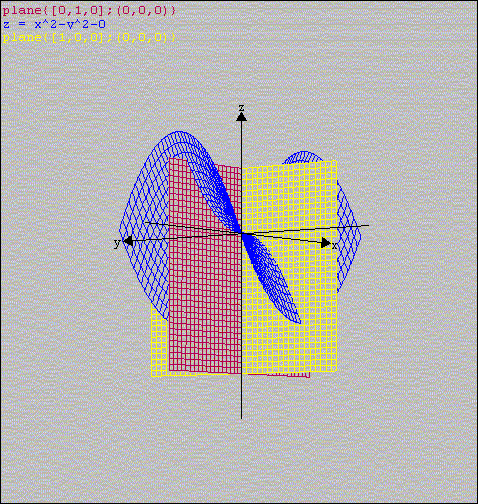# Why quadratic equation may have complex solutions?

Anywhere you read you will learn that when you calculate the discriminant (the expression inside the square root) and if it is greater than 0 then you have two solutions, when it is equal to 0 than you have two equal solutions, but if it is less than 0 then there are no solutions among real numbers.
They may also explain that "if you really want to", then the solutions in the latter case can be found among complex numbers.
I, too, learned this at school and in college and never thought twice about it until my son asked me to explain.
This is when I realized I really don't quite understand it myself even being M.S. in applied Math and Computer Science.
After a few weeks of asking around and with a big help from my friend Boris Agranovski I finally understood it.
In order to follow my explanation you need to know at least basics about complex numbers.
You can check Purplemath: Complex Numbers or get a very detailed and relatively boring explanation of compelx numbers at Wikipedia - complex numbers.
You can also learn the "official" version about the formula for solving a quadratic equation also at Purplemath: The formula explained
or at Wikipedia - quadratic formula

## Simplified case

Let's look at the behavior of a simplified quadratic formula:
 z = x2 + p (1)

For reasons you will see very soon I chose variable z instead of usual y.
The more complex formula
 z = a * x2 + b*x + c
behaves the same way, but it is more difficult to graph since it is not centered around axis z, so we will stick to the simple formula (1).
Let's see how solutions behave when in formula (1) we try different values of p. We will try values p=-4, p=-1, p=0, p=1, and p=4 (obviously, when p > 0 there are no solutions in real numbers and this is where we are supposed to switch to complex numbers).Back Next

As one can see, as the parabola moves up (p grows) the solutions move closer and eventually become one value and then all of a sudden the solutions move to an imaginary numbers which are represented on axis y and start moving away from each other. Isn't it a bit odd? When p=+1 you may think, that the solution may be somehow related to the line (as one of the solutions at least lies on the parabola), but when parabola moves higher, this hope dissipates - the solutions in complex numbers don't seem to have anything to do with the parabola anymore.

## Parabola for complex numbers

Let's write a quadratic function of a complex variable. For one moment call this variable xc.
 z=xc2+p (2)

Then considering that xc=x+iy we will get
z=xc2+p=(x+iy)2+p=x2+2xyi+(iy)2+p= {since i2=-1} =x2+2xyi-y2+p=x2-y2+p+2xyi

So, the simplified version is
 z=x2- y2+p+2xyi (3)

Function (3) is the one we are going to look at.
In fact we will simplify it even further:
As you can see the expression for z consists of two parts - real (x2- y2 + p) and imaginary (2xy). We are only going to deal with the real part of it. So, finally, the function we are going to deal with is
 z=x2- y2 + p (4)

## Real part of a parabola for complex numbers

Let's try to draw this function (remember, this is a 3D world now) and start with, say, p=-4Back Next

Well, it turns out that the shape of this function is a saddle!

## Parabola number 1

Now, our original equation (1) corresponds to equation (4) when y=0 (which is a plane that includes axis X and axis Z).
Here it is:Back Next

As you can easily see at this figure, when y=0, we have z=x2 - 4 and its graph has a shape of a parabola on a plane xz. This is the parabola we usually study at school.
You can also see that it has two solutions x=-2 and x=+2 where z=0. (Please remember that the figure you see is not in proper scale in order to make a 3D figure look better). We are used to drawing parabola pictures on paper, so the parabola you see drawn blue on red at the picture above is what you usually draw at school.

## Parabola number 2

But wait, what if we look at the other plane (x=0)? What can we make out of a plane x=0 intersecting with our saddle? Let's see:Back Next

So, we can see that when x=0 our equation (4) turns into z=-y2 - 4 and is also a parabola, but this time it is open downward and never crosses line z=0.

## Two parabolas

Now, what do you think will happen if we start moving the saddle up? It corresponds to making coefficient p bigger. Let's try p=-1, p=0, p=1, p=4.
So, this one is for p = -1:Back Next

This one is for p = 0:Back Next

OK, p = +1:Back Next

And finilly, p = +4Back Next

So, here is what is happening: the parabola we are studying at school is represented by interception of a blue saddle and a red plane. It really corresponds to our equation (4) when y=0 (hence it all lies in the red plane). When p grows and becomes positive, the parabola created by intersection of the blue saddle and the red plane moves above surface z=0.
In the same time look at the parabola created by intersection of the saddle and the yellow plane. It represents equation (4) when x=0 (which means it consists of imaginary numbers only) and the equation becomes
z= - y2 + p
When p was less than 0 the entire parabola lay below line z=0. But as p grows to be greater than 0 its top now lies above the line z=0 and since it opens down it will start intercepting the line z=0.

## Conclusion

When we are solving the equation
z = x2 + p
we, in fact, are also keeping track of a related equation
z = - y2 + p
and when we can't find solutions of the original parabola we are switching to solving the related equation and announcing it to be "complex" solutions. I think the reason for this is that it seems to be a convinient place to either introduce or at least demonstrate the complex numbers, that otherwise look very odd in school curricculum.